# 空间域图像增强

Posted by jjx on December 22, 2016

• 空间域滤波的基础知识
• 相关和卷积
• 图像平滑， 包括平均平滑和高斯平滑

### 图像增强基础

g(x,y)=T[f(x,y)]

#### 空间域滤波

(1) 对预先定义的以（x, y） 为中心的邻域内的像素进行运算。
(2）将（2）中运算的结果作为（x, y）点的新响应。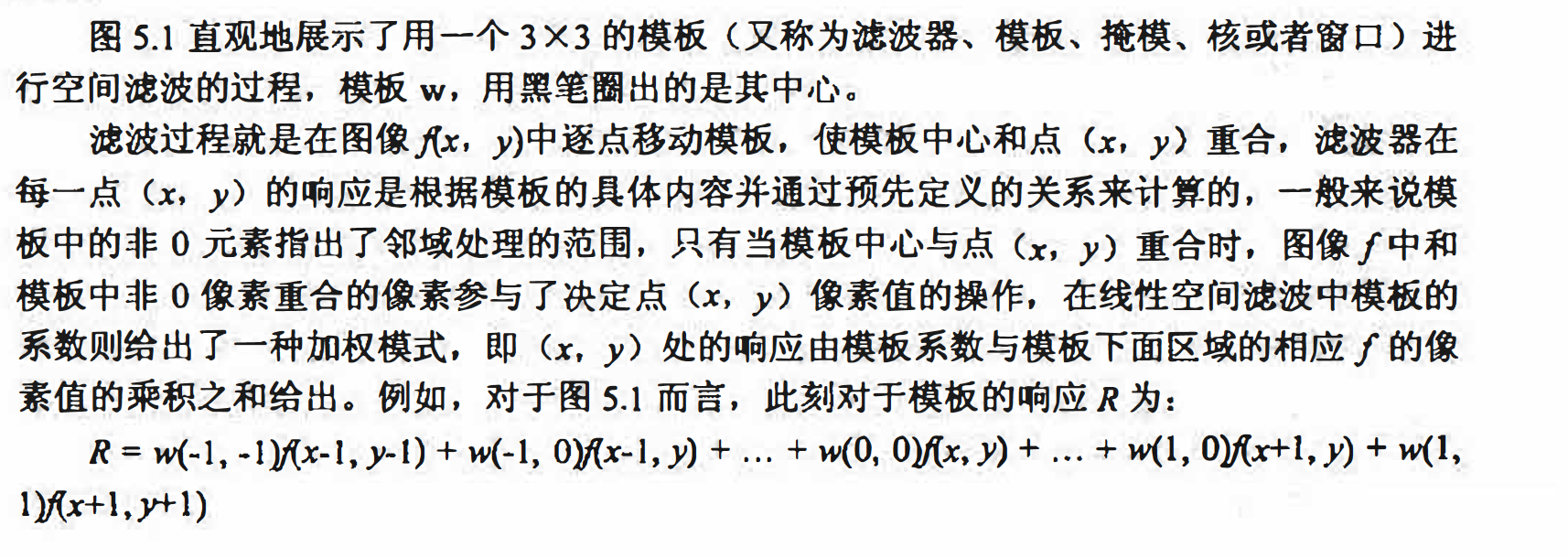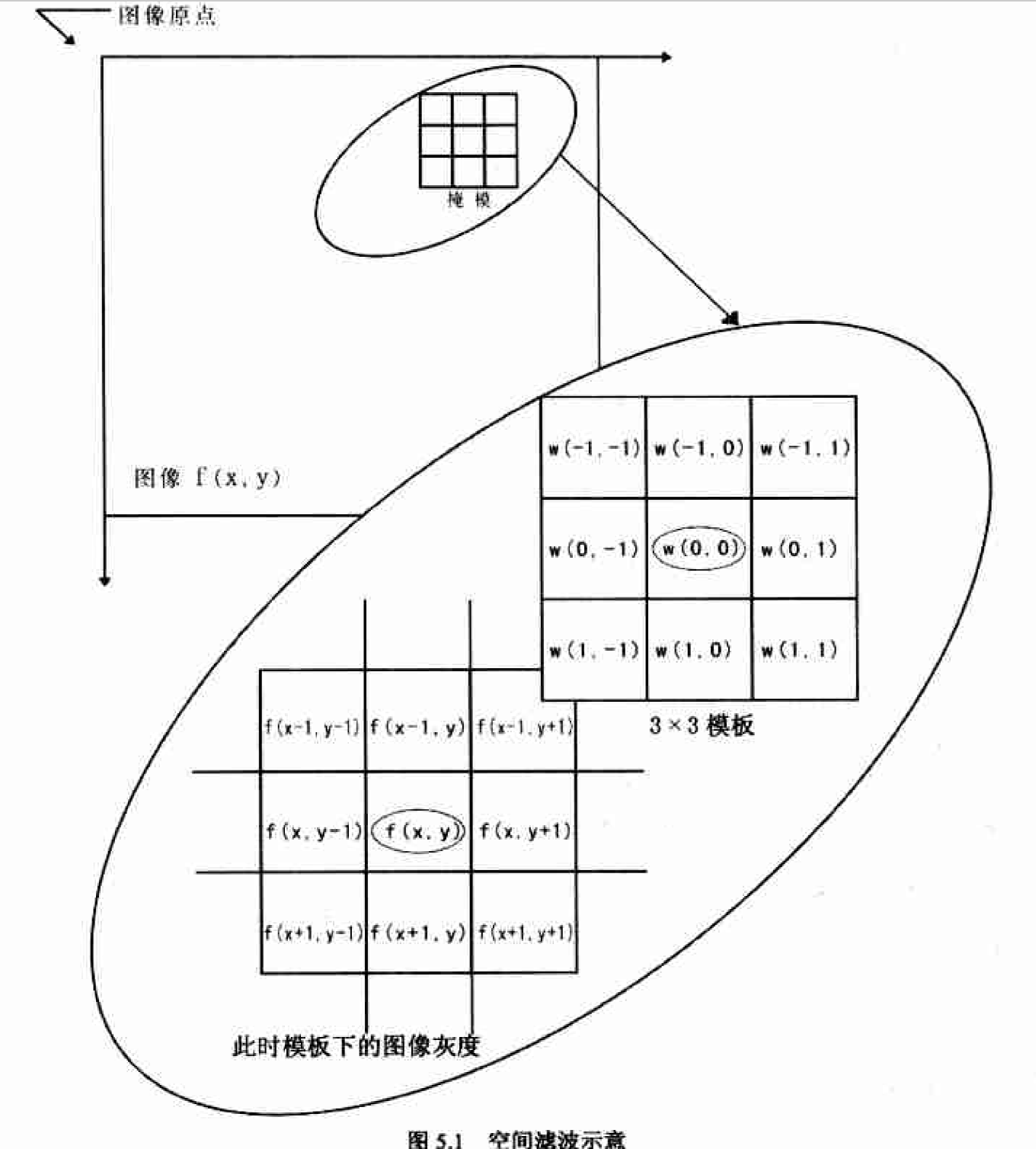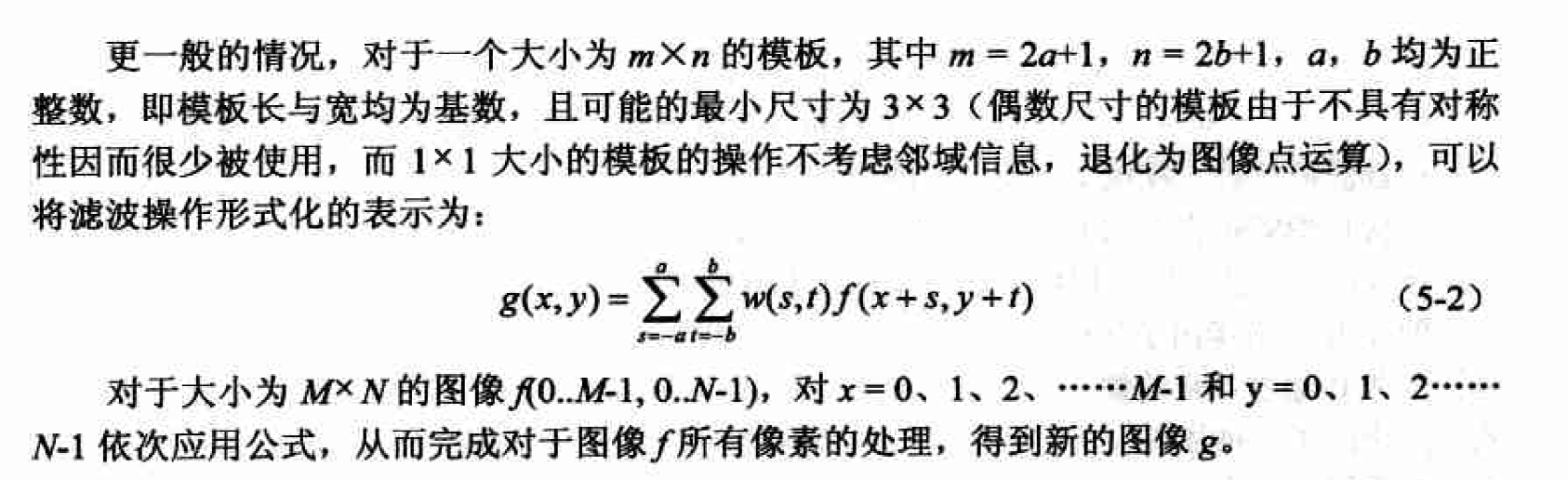(1）收缩处理范围一处理时忽略位于图像f 边界附近会引起问题的那些点， 如对于 图5.1中所使用的模板， 处理时忽略图像f 四周一圈1个像素宽的边界， 即只处理从x = 1，2 ,3, … .M-2和y = 1,2,3, .. .N-2 （在Matlab中应为x = 2,3,4, … ,M-1和y = 2,3,4, … N-1）范围内 的点， 从而确保了滤被过程中模板始终不会超出图像f 的边界。
(2）使用常数填充图像一根据模板形状为图像f 虚拟出边界． 虚拟边界像素值为指定 的常数， 如0， 得到虚拟图像f ’。保证模板在移动过程中始终不会超出f ’的边界。
(3）使用复制像素的方法填充图像,和（2）基本相同， 只是用来填充虚拟边界像素 值的不是固定的常数， 而是复制图像f本身边界的模式．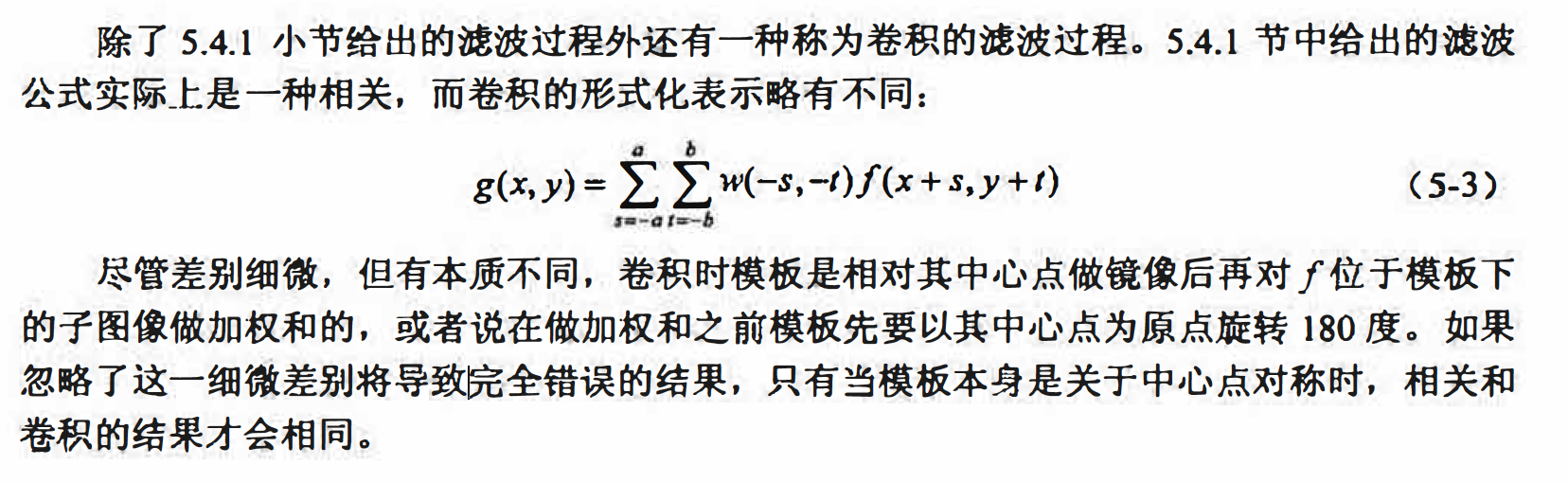Matlab中与滤披相关的函数主要有imfilter和fspecial. imfilter完成滤波操作，而fspecial 可以为我们创建一些预定义的2维滤波器， 直接供imfilter函数使用．

g =imfilter(f,w,optional1,optional2);

• f是要进行滤波操作的图像． • w是滤波操作所使用的模板，为一个二维数组．
• option 1, option2, … 是可选项， 具体可以包括：

(1）边界选项： 主要针对5.2.2 小节中提到的边界处理问题， 如表5.1所示。 采用第一种方式用固定值填充虚拟边界会使边缘附近会产生梯度， 采用后面三种方式填 充可让边缘显得平滑。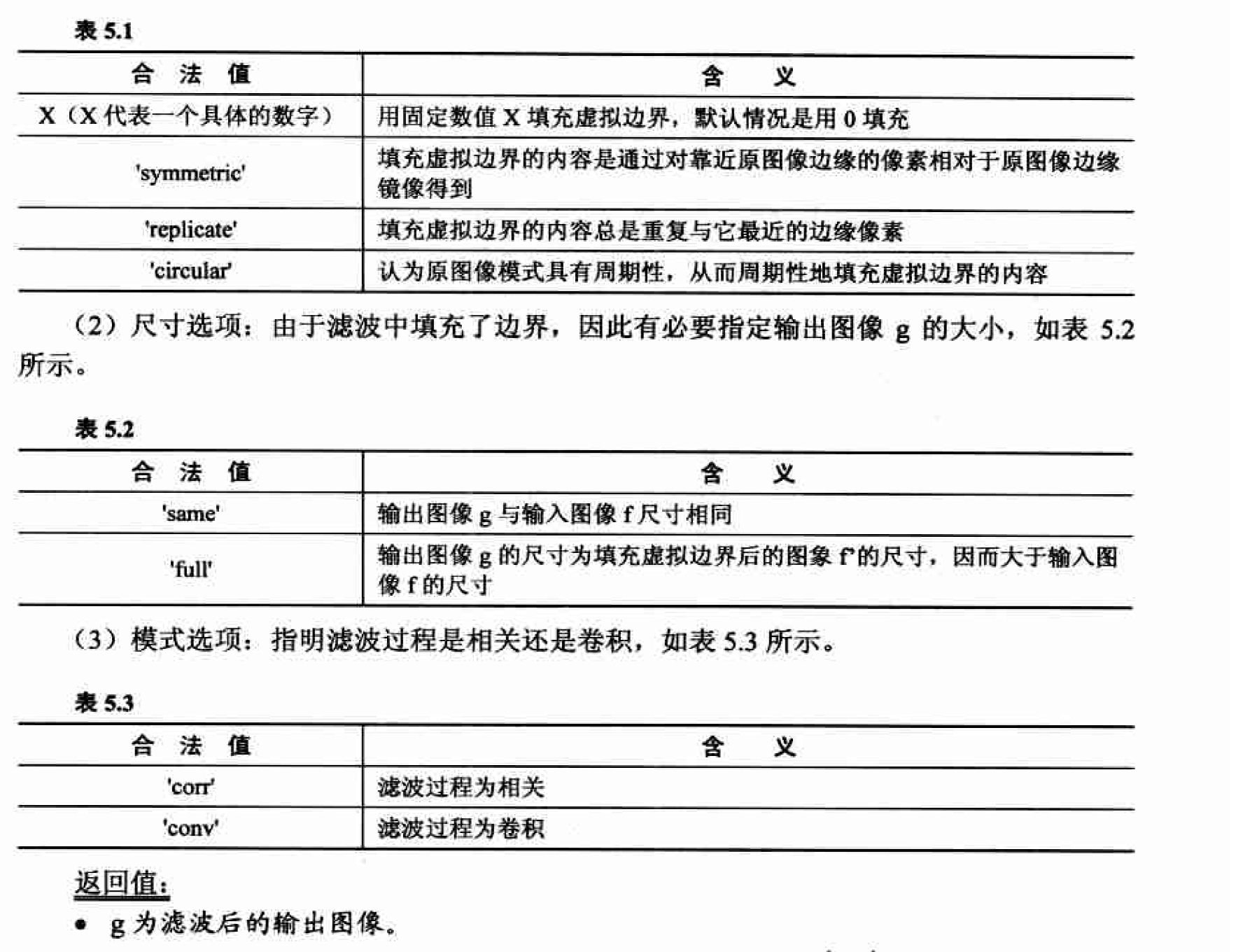f = imread('cameraman.tif');
w = [1,1,1;1,1,1;1,1,10]/9;
g = imfilter(f,w,'corr','replicate');
figure;
subplot(1,2,1);
imshow(f),title('原图像');
subplot(1,2,2);
imshow(g),title('滤波操作');fspecial创建预定义的二维滤波器

h = fspecial(type,paramaters)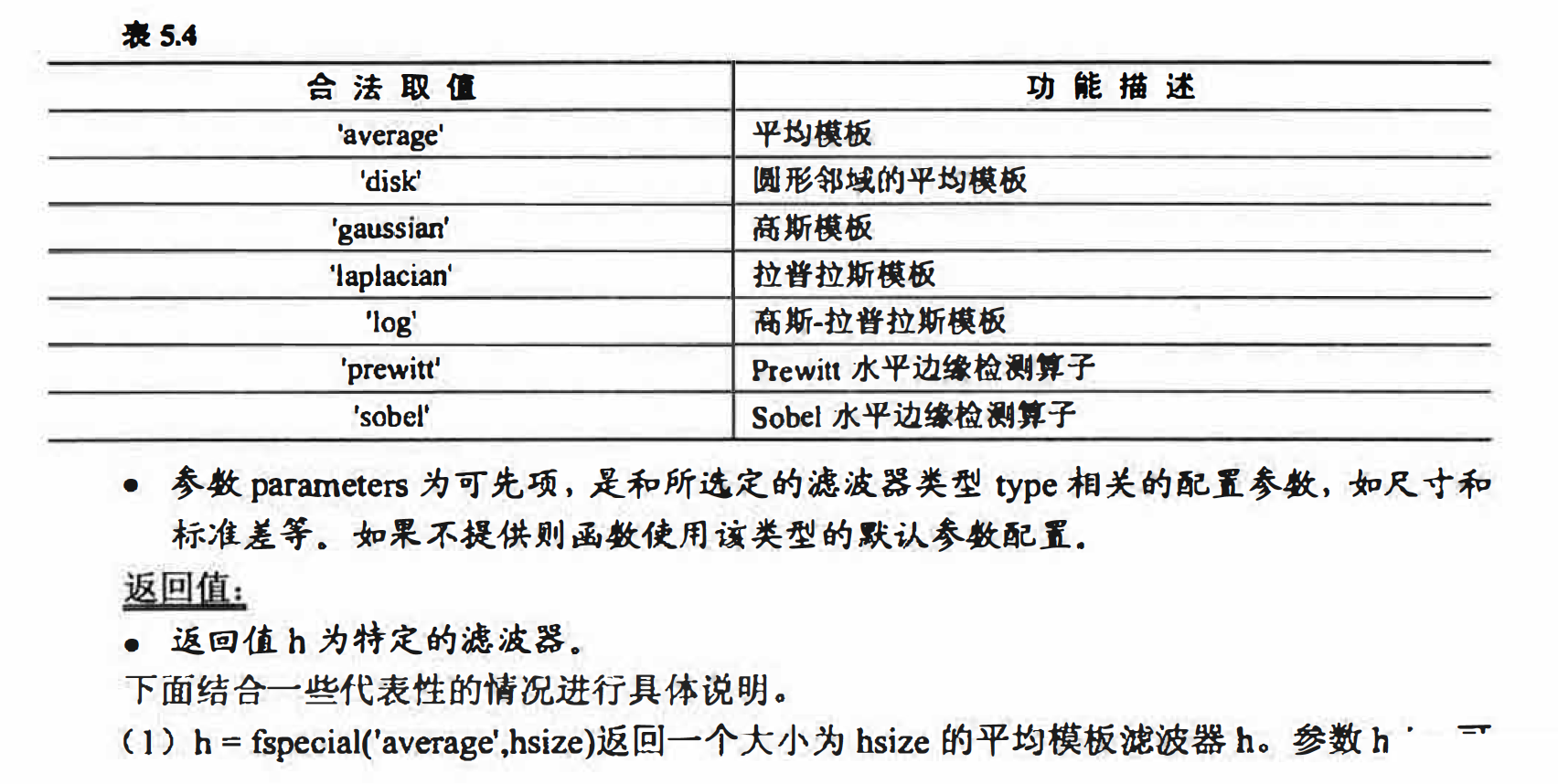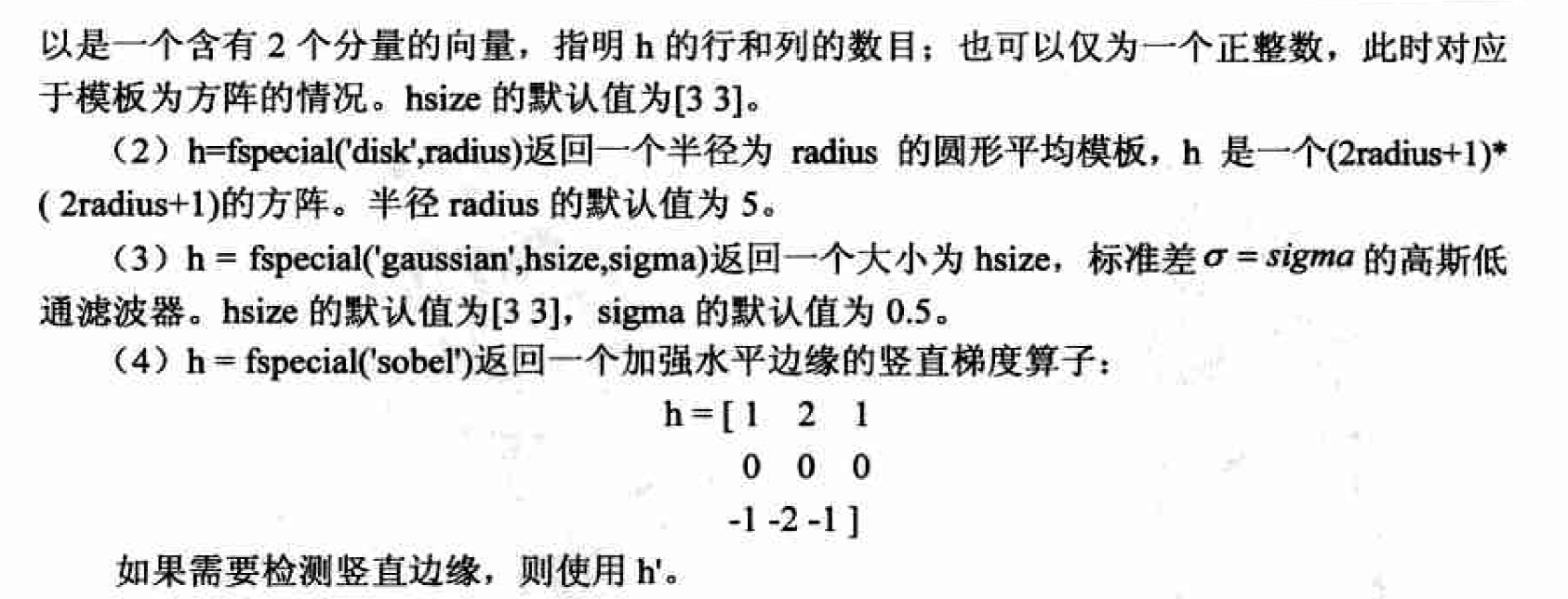### 图像平滑

#### 平均模板及其实现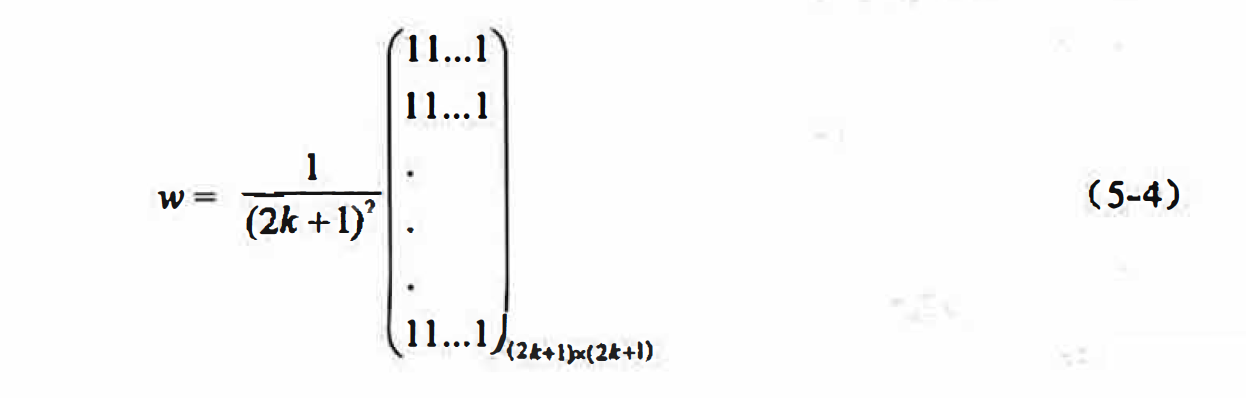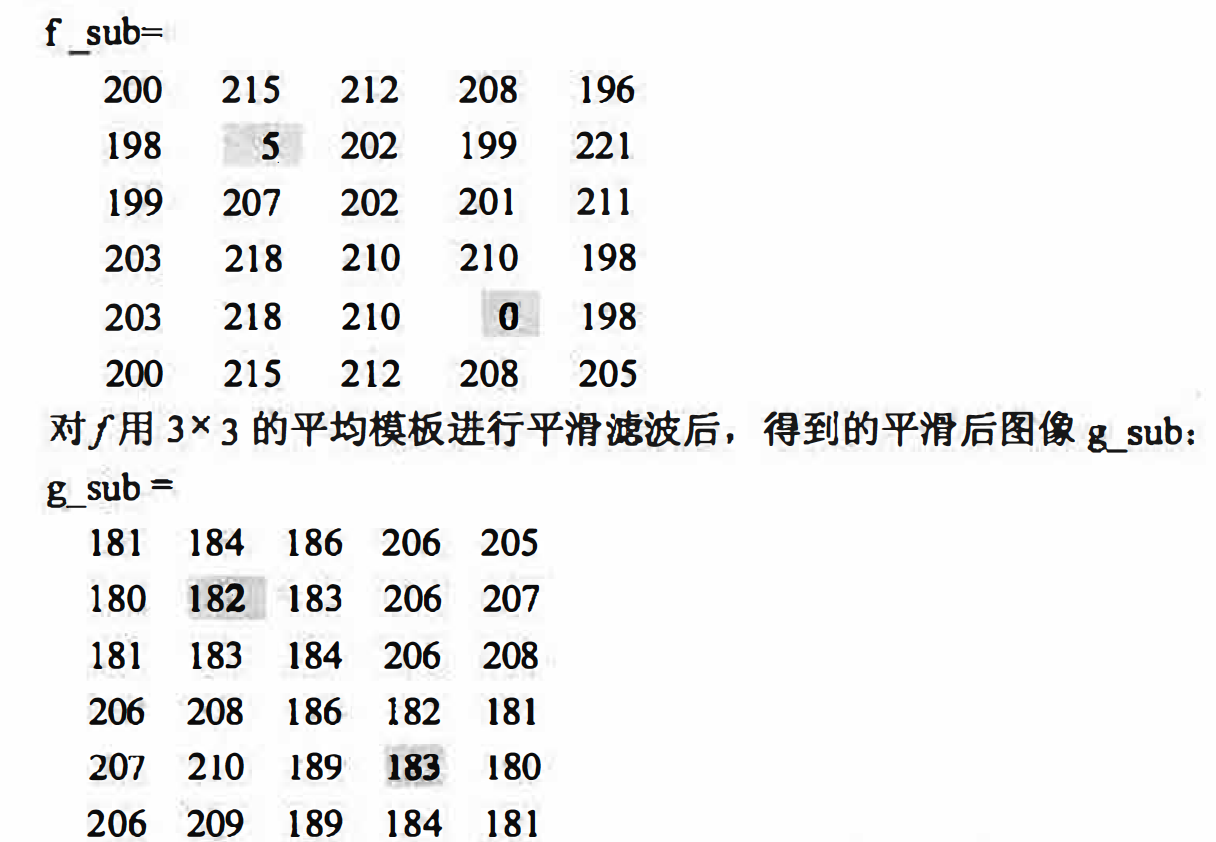Matlab实现

I = imread('baby_noise.bmp');
h = fspecial('average',3);
I3 = imfilter(I,h,'corr','replicate');
h = fspecial('average',5);
I5 = imfilter(I,h,'corr','replicate');
h = fspecial('average',7);
I7 = imfilter(I,h,'corr','replicate');
figure;
subplot(2,2,1);
imshow(I),title('原图');

subplot(2,2,2);
imshow(I3),title('3*3');
subplot(2,2,3);
imshow(I5),title('5*5');
subplot(2,2,4);
imshow(I7),title('7*7');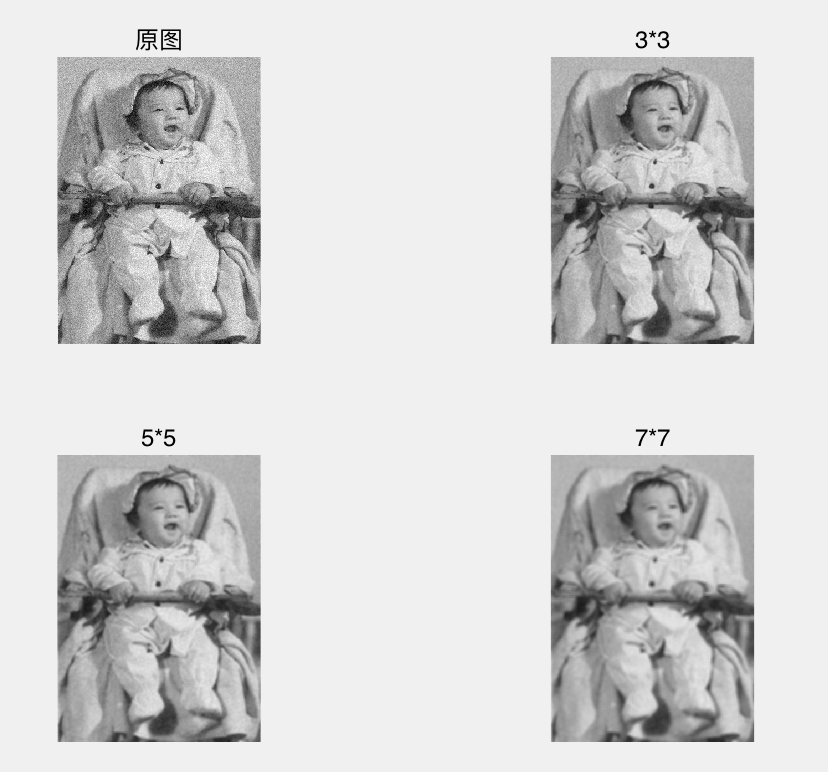#### 高斯平滑及其实现

matlab实现

I = imread('baby_noise.bmp');
h3_5 = fspecial('gaussian',3,0.5);
I3_5 = imfilter(I,h3_5);

h3_8 = fspecial('gaussian',3,0.8);
I3_8 = imfilter(I,h3_8);
h3_18 = fspecial('gaussian',3,1.8);
I3_18 = imfilter(I,h3_18);

h5_8 = fspecial('gaussian',5,0.8);
I5_8 = imfilter(I,h5_8);

h7_12 = fspecial('gaussian',7,1.2);
I7_12 = imfilter(I,h7_12);

figure;
subplot(2,3,1);
imshow(I);
subplot(2,3,2);
imshow(I3_5);
subplot(2,3,3);
imshow(I3_8);
subplot(2,3,4);
imshow(I3_18);
subplot(2,3,5);
imshow(I5_8);
subplot(2,3,6);
imshow(I7_12);


#### 自适应平滑滤波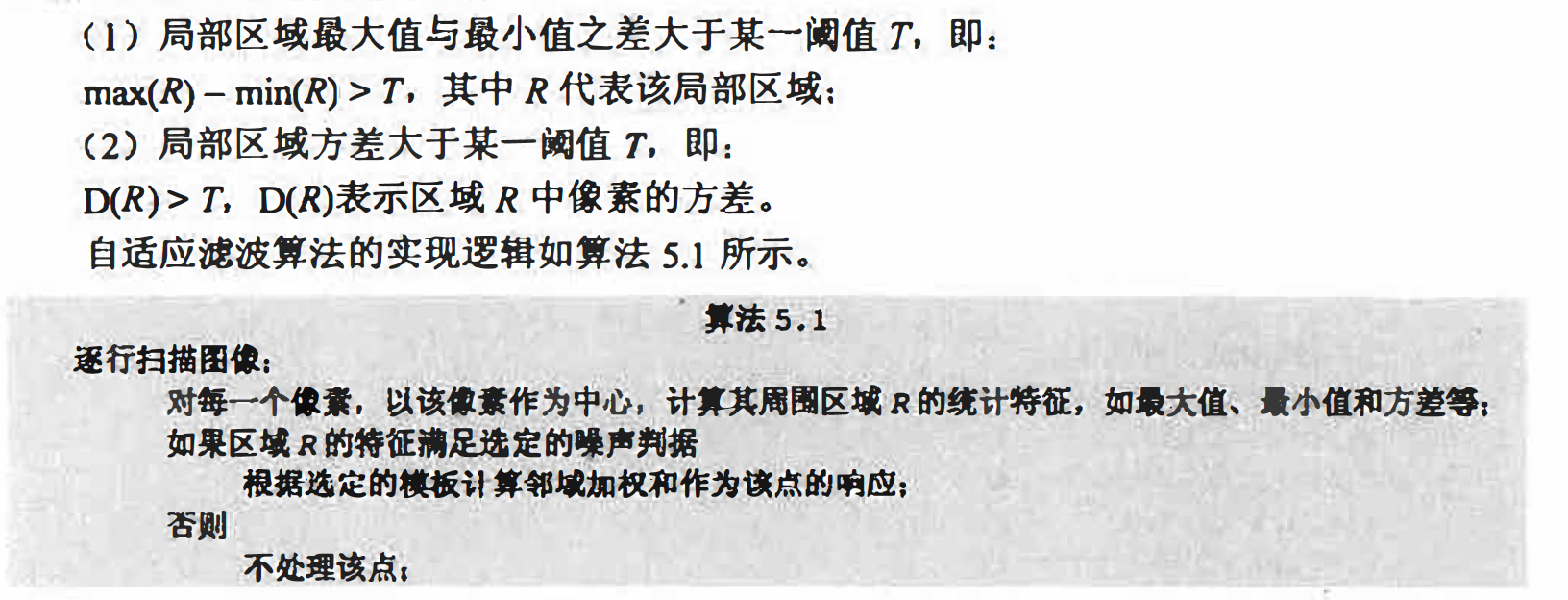对于那些噪声位置具有随机性和局部性的图像， 自适应的滤波具有非常好的效果． 有兴 趣的读者可自己编制程序实现自适应的高斯平滑算法， 应用于具有上述特点的噪声图像中， 并且，和我们给出的标准高斯平滑效果进行比较．